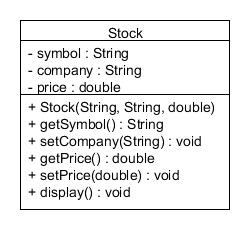## ArrayList with FOR Loop

There are many common questions related to use of FOR loop in going through elements in ArrayList. Let's give an example:Question: We have an ArrayList stockList containing Stock objects. Calculate the sum of prices of all the elements and display the sum.

Following the steps given in the slides, we need to

1. Declare a variable to sum up all values and initialise it to 0
2. Go through each value and add it to the variable
3. Print out the variable after the FOR loop

Step 1:

For step 1, think about what data type we should use for the variable, it should be the same as the values we need to sum up. In this case, price is of type double, so the sum should be of type double as well.

double sum = 0;

Step 2:

Step 2.1 To go through the ArrayList, we need to use a FOR loop. A common structure is like this:

for (int a = 0; a < arrayListVariable.size(); a++) {
//Statements
}

So to apply it to our example,

for (int a = 0; a < stockList.size(); a++) {
//Statements
}

Inside the FOR loop, think about how to get the price of each element and add them together.

Step 2.2  To get the element, from there, you try to get the desired field (in our case price) of the element. Still remember how to get an element of an ArrayList? we use arrayListVariable.get(i) where i refers to the position of the element. So in this case, we use stockList.get(a)

Step 2.3 To get the price of the element, we look at the getter methods available, which one should we use? Obviously getPrice().

Step 2.4 To add to the variable we use to sum up the all values, we should add it to the variable, and assign the total prices to the variable again.

common structure:

sum = sum + value of one element

Now we combine everything for step 2

for (int a = 0; a < stockList.size(); a++) {
sum = sum + stockList.get(a).getPrice();
}

Step 3:

At the end, we should print out the sum to the screen. To display the value, always think about what is the data type of the value, it is a reference type (Car object, Employee object, String, etc) or primitive types (int, double) . For primitive type and String, we usually use System.out.println directly, whereas for others, we need to look for the method available for the object class to find out the method to use (some common names are display(), show(), showInfo(), printInfo() )

In our case, the data type of sum is double, so we should use System.out.println().

System.out.println("the sum of prices of all elements is "+ sum);

Putting All Together:

double sum = 0;
for (int a = 0; a < stockList.size(); a++)
{
sum = sum + stockList.get(a).getPrice();
}
System.out.println("the sum of prices of all elements is "+ sum);

Practice Question: Find out the Stock object with the max price in the ArrayListstockList, and display the object.

Hint: Think about the steps you need to do in order to solve the question,  some standard steps can be found here.

Solution:

Stock maxStock = stockList.get(0);  //initialize maxStock to be the first element of the ArrayList
for (int a = 0; a < stockList.size(); a++)
{
if(maxStock.getPrice()< stockList.get(a).getPrice()) //compare the price of maxStock with the current element
{
maxStock = stockList.get(a); //if the current element has higher price, update the maxStock to be the element
};
}
maxStock.display(); //display the object, since it is of type Stock, cannot use System.out.println(), instead, should look into the class diagram to find out the correct method to use